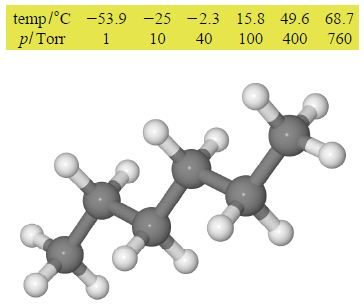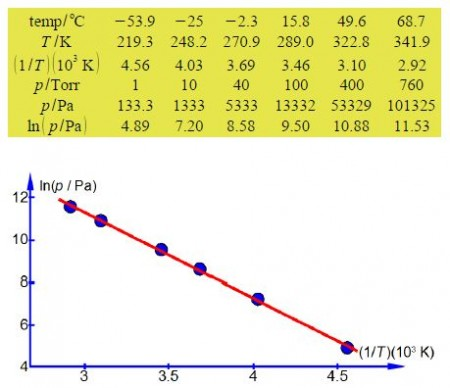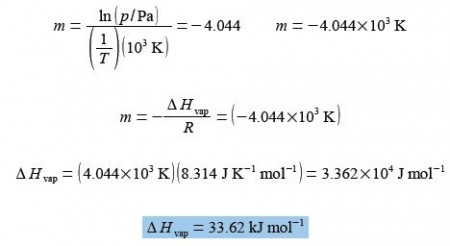# How to Calculate Vapour Pressure Data for Liquid?

The vapour pressure of the solvent above a liquid varies with temperature according to

ln(p) = – [ΔHvap/ RT] + B

where p is the vapour pressure; ΔHvap is a measure of the energy required to evaporate a mole of the solvent (that is transform it from liquid to its gaseous form); T is the temperature in kelvin; and B is a constant. A student in the teaching lab obtained the following data which lists the measured vapour pressure of hexane in torr at various temperatures in °C up to its boiling point at 68.7°C. A torr (symbol Torr) is a convenient unit of pressure when using a mercury manometer with a ruler calibrated in millimetres even though it is non-SI (760 Torr = 101325 Pa = 1 atm). The temperatures are converted using 0°C = 273.15 K. Graphically determine ΔHvap.Here,

In order to have a straight line graph we construct a table for ln(p/Pa) and (1/T)(103 K).The straight line that I measured from the full sized manually drawn graph is y = -4.044x + 23.43 which taking into account the labels of the axes, including the unit multiples, gives the enthalpy of vaporization asThe student’s measured value is higher by 16% than the accepted literature value of 28.85 kJ mol-1 and the method and apparatus both need careful evaluation.